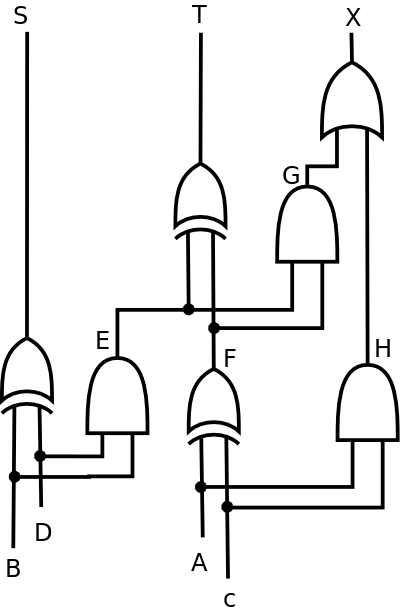# Assignment 2

Due Monday 1 October in class.

1. Write your first name in binary and hexadecimal, using the ASCII encoding. Here is mine, as an example:

``````C        h        r        i        s         (text)
67       104      114      105      115       (decimal)
01000011 01101000 01110010 01101001 01110011  (binary)
43       68       72       69       73        (hex)``````
2. Decode the following message. Each chunk is an 8-bit binary number representing the ASCII code for a letter.

``01010011  01110100  01110101  01100100  01011001  00100001``
3. Use truth tables to prove the equivalence of these two Boolean expressions:

``    X·Y + X'·Z + Y·Z  =  X·Y + X'·Z``
4. Below is a reasonably complex combinational circuit diagram. It contains three XOR gates, three AND gates, and one OR gate. It has four inputs (labeled A, B, C, D – the strange order is intentional), three outputs (labeled X, T, S), and four intermediate wires (labeled E, F, G, H). Make sure you can find all those components before moving on.